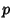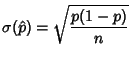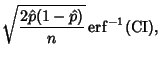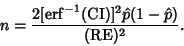## Sample Proportion

Let there besuccesses out ofBernoulli Trials. The sample proportion is the fraction of samples which were successes, so(1)

For large,has an approximately Normal Distribution. Let RE be the Relative Error and SE the Standard Error, then(2)(3)(4)

where CI is the Confidence Interval andis the Erf function. The number of tries needed to determinewith Relative Error RE and Confidence Interval CI is(5)